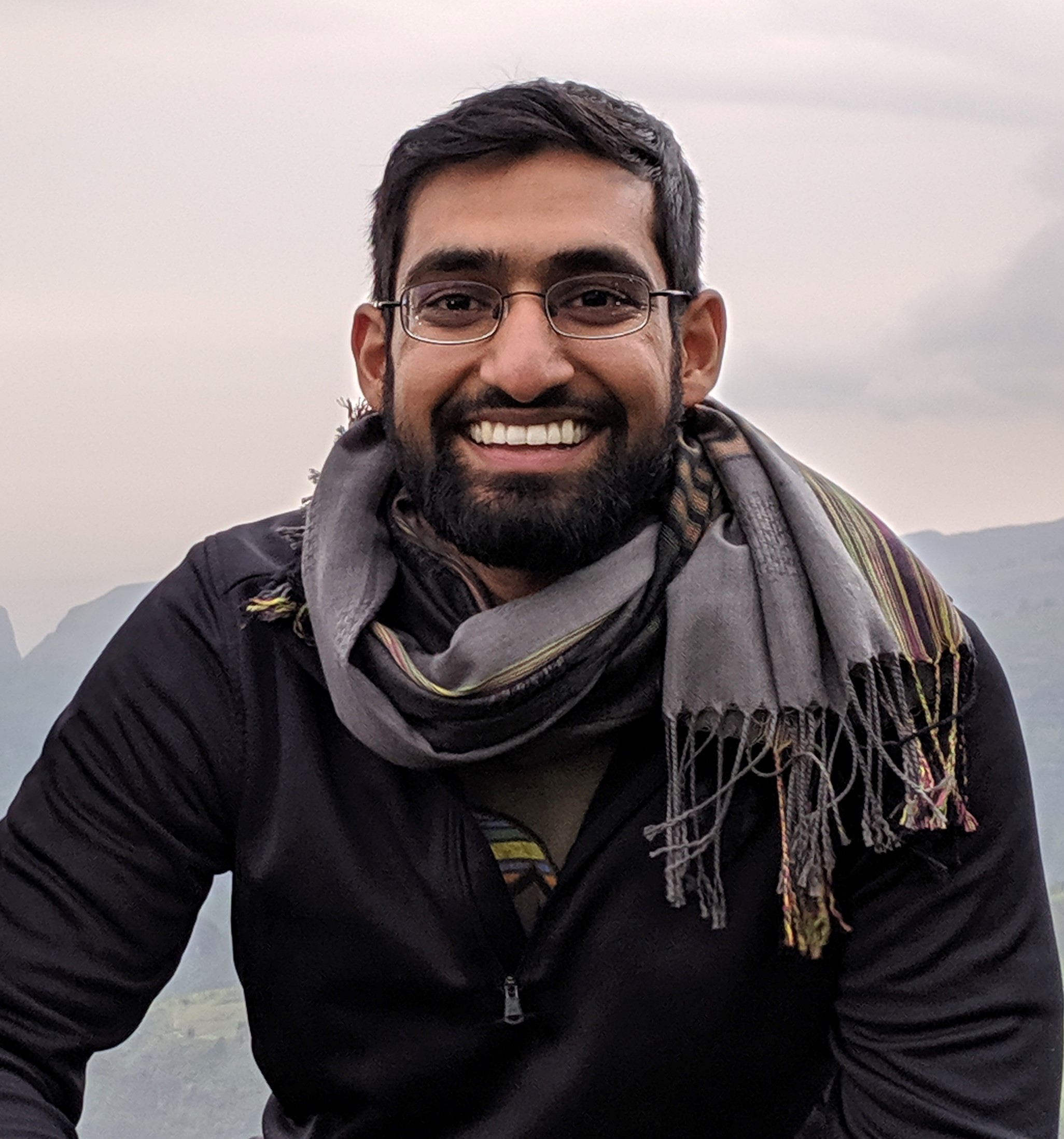# Applied Bayesian Data Analysis for Research / Spring 2023

• 01/08 -- New Lecture is up: Lecture 5 -- How are Bayesian models fit? Part 2 [slides]
• 01/08 -- New Lecture is up: Lecture 4 -- How are Bayesian models fit? Part 1 [slides]
• 01/08 -- New Lecture is up: Lecture 3 -- Priors, multivariate models, regression [slides]
• 01/08 -- New Lecture is up: Lecture 2 -- Bayesian analysis introduction – single parameter models [slides]
• 01/08 -- New Lecture is up: Lecture 1 - Course Introduction [slides]
• 01/18 -- New Assignment released: [Paper presentation and replication]
• 01/18 -- New Assignment released: [Homework #1]

## Course Description

Bayesian modeling and data analysis is a powerful tool for computational research. It consists of writing a probability model and then fitting it with observed data, while handling uncertainty. The model can be flexible, encompassing hierarchy, spatio-temporal dynamics, graphs, and high-dimensionality. This course is a graduate, hands-on introduction to Bayesian analysis in Stan and/or Pyro. The focus will be on writing and fitting models in practice for computational research, including the applied Bayesian statistics workflow: model building, checking, and evaluation. The course will also discuss research papers that use such methods.

See the syllabus for details. The class will be hybrid remote, with students split across Ithaca and NYC.

Course topics

• Introduction and overview of Bayesian inference (~1 weeks)
• Developing and debugging basic models in Stan/Pyro (~2 weeks)
• Single parameter models, multivariate models, Bayesian regression, Multinomial choice models, Hierarchical models
• Basics of sampling algorithms
• Bayesian workflow (~1-2 weeks)
• Model checking (posterior predictive checks, diagnosing identifiability issues, etc)
• Evaluating, comparing models
• Research paper case studies (~4-5 weeks)
• Each lecture we will discuss 1 paper. There will be student presenters for the paper (via slides), and then we will replicate in code the model + fitting from the paper.
• Miscellaneous topics (~1-2 weeks)
• Some guest lectures
• Advanced models – gaussian processes/dynamic models, HMMs and Spatial models, conditional random fields, nonlinear and nonparametric models
• Sampling algorithms (Gibbs, Metropolis Hastings, HMC, Variational Inference)
• Computation concerns (speeding up models, GPU usage, etc)
• Student project presentations (1-2 weeks)

## InstructorNikhil Garg